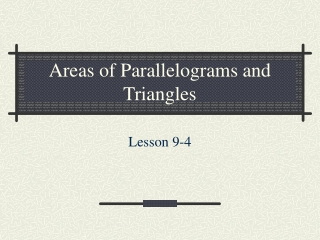DownloadDownload PresentationAreas of Parallelograms and Triangles

# Areas of Parallelograms and Triangles

Download Presentation## Areas of Parallelograms and Triangles

- - - - - - - - - - - - - - - - - - - - - - - - - - - E N D - - - - - - - - - - - - - - - - - - - - - - - - - - -
##### Presentation Transcript

1. Areas of Parallelograms and Triangles Lesson 9-4

2. Parallelogram • A parallelogram is a quadrilateral where the opposite sides are congruent and parallel. • A rectangle is a type of parallelogram, but we often see parallelograms that are not rectangles (parallelograms without right angles).

3. Area of a Parallelogram • Any side of a parallelogram can be considered a base. The height of a parallelogram is the perpendicular distance between opposite bases. • The area formula is A=bh A=bh A=5(3) A=15m2

4. Area of a Triangle • A triangle is a three sided polygon. Any side can be the base of the triangle. The height of the triangle is the perpendicular length from a vertex to the opposite base. • A triangle (which can be formed by splitting a parallelogram in half) has a similar area formula: A = ½ bh.

5. Example A= ½ bh A= ½ (30)(10) A= ½ (300) A= 150 km2

6. Complex Figures • Use the appropriate formula to find the area of each piece. • Add the areas together for the total area.

7. Example 24 cm 10 cm | 27 cm | Split the shape into a rectangle and triangle. The rectangle is 24cm long and 10 cm wide. The triangle has a base of 3 cm and a height of 10 cm.

8. Solution Rectangle Triangle A = ½ bh A = lw A = ½ (3)(10) A = 24(10) A = 240 cm2 A = ½ (30) A = 15 cm2 Total Figure A = A1 + A2 A = 240 + 15 = 255 cm2

9. Homework Time